# Problem 57646. Easy Sequences 93: Recursive Polynomial Function

For a natural number x and a polynomial function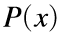, we define a recursive function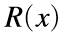, as follows: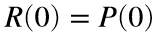, and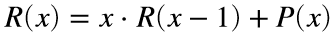, for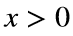.
For example, if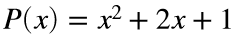(or in Matlab array form, P = [1 2 1]):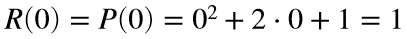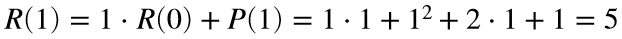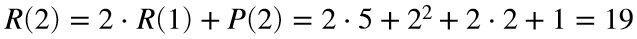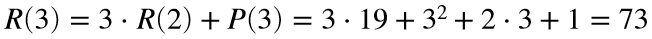...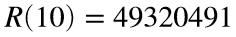and so on...
We can see thatcan grow very quickly. Therefore, we will instead calculate: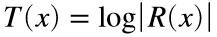, that is,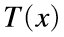is equal to the logarithm (base-e) of the absolute value of.
Given a polynomial array P, and an integer x, find the value of, rounded-off to 4 decimal places.
----------------------
NOTE: To encourage vectorization , FOR and WHILE loops are disabled. If you know the math, this problem can be solved in less than 15 lines of code. However, solutions up to 50 lines of code will still be accepted. The semicolon (;), shall be considered as an end-of-line character.

### Solution Stats

66.67% Correct | 33.33% Incorrect
Last Solution submitted on Apr 29, 2023

### Community Treasure Hunt

Find the treasures in MATLAB Central and discover how the community can help you!

Start Hunting!